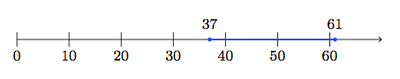# Height Requirements

Alignments to Content Standards: 6.EE.B.8

At Sea World San Diego, kids are only allowed into the Air Bounce if they are between 37 and 61 inches tall. They are only allowed on the Tide Pool Climb if they are 39 inches tall or under:1. Represent the height requirements of each ride with inequalities.

2. Show the allowable heights for the rides on separate number lines.

3. Using inequalities and a number line, describe the height of kids who can go on the both the Air Bounce and the Tide Pool Climb.

## IM Commentary

The goal of this task is to express constraints from a real world context using one or more inequalities. In addition to writing inequalities, students also display the numbers (heights) satisfying the inequalities on a number line. Students could represent the height, h, in inches of a passenger on the Air Bounce with the inequalities $h \gt 37$ and $h \lt 61$ (or with the compound inequality 37 $\lt h \lt$ 61, though this compound notation is not required in grade 6). The language 'between' 37 and 61 inches' can correctly be interpreted this way. In the solution we included the end points, 37 inches and 61 inches. Given the sign for the Tide Pool Climb, this is likely how 'between' is being used for the Air Bounce.

The context for the problem creates some subtle issues which the teacher may need to address. Heights are often recorded to the nearest inch. So for the Air Bounce ride, students might provide a list of possible heights: on the number line, this would correspond to marking just whole numbers from 37 up to 61. The number line model should help with this confusion, however, as the teacher can ask students if someone who is, for example, 42$\frac{1}{4}$ inches could go on the Air Bounce ride. Rounding to the nearest whole number creates an additional problem: with rounding, a child who is 36$\frac{1}{2}$ inches tall could go on the Air Bounce which disagrees with the statement on the sign.

## Solution

1. For the Air Bounce, children must be at least 37 inches tall. If we let $h$ denote the child's height in inches, this means $h \geq 37$. They are also not allowed to be more than 61 inches tall so $h \leq 61$. We say $h \geq 37$ and $h \leq 61$. (This can be written as a compound inequality, but that is not expected at grade 6)

For the Tide Pool Climb, children are not allowed to be over 39 inches. Using $h$ for the child's height, this is represented by $h \leq 39.$ We should also write this as $0 \lt h$ since a height cannot be zero or negative.

2. The allowable heights in inches for Air Bounce are shaded blue on the number line below (note that they include 37 inches and 61 inches). No negative numbers are included on the number line as this does not make sense for the context of height.The allowable heights for Tide Pool Climb are shaded purple on the number line below:Although it is not possible for a child to be close to 0 inches tall, these numbers are shaded because they fit the inequality $h \leq 39$. No negative numbers (or 0) are plotted because they do not make sense in the context of height. So the graph shows heights satisfying $0 \lt h$ and $h \leq 39$.

3. In order go on the Tide Pool Climb, a child cannot be over 39 inches in height. In order to go on the Air Bounce, a child has to be at least 37 inches tall but not more than 61 inches. So to go on both, a child must be at least 37 inches tall but not more than 39 inches: $37 \leq h$ and $h \leq 39.$ These heights in inches are highlighted on the number line below:Notice that the shaded parts of this number line are where the shaded parts of the two previous underlines overlap: this makes sense as we are looking for heights of those who can go on both rides.